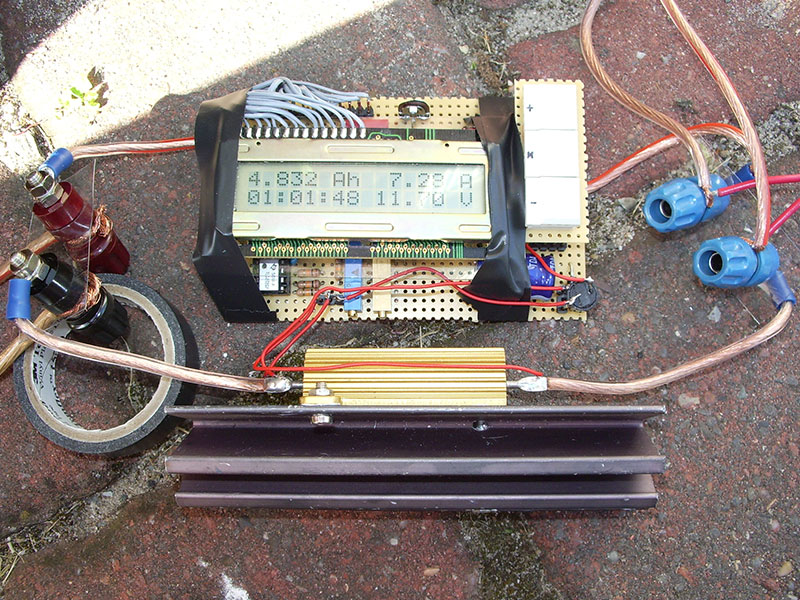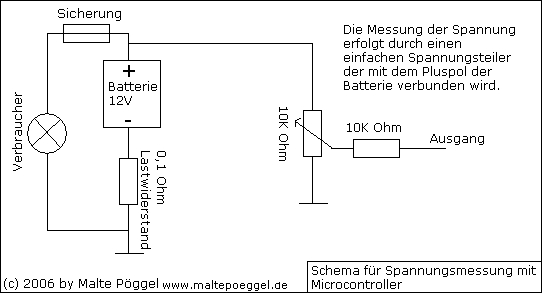### Battery capacity meter

Measures the capacity of 12V batteries

For tinkering and mobile power supply I have an older car battery in the garage. But now the question came up, what capacity it has left at all. The cheapest method would be to connect a lamp and stop the time until the battery has reached the final discharge voltage. However, if you take into account that the voltage (and thus the load) decreases during the discharge process, this method is quite inaccurate.So something better is needed. A microcontroller that monitors and calculates all values seems to be more suitable.

The only raw data we need to measure and process are current and voltage. In addition a time measure is needed which the controller generates itself. (per second)

The milliampere hours that have to be added per second (more precisely: milliampere seconds) are calculated from the formula I (current in mA) / 60 / 60. So with a discharge current of 1000mA, 0.27 mA would be taken per second.

The measurement circuit looks as follows:Depending on the current, the voltage at the output increases. The voltage is later converted to a digital value by means of an analog-to-digital converter.Voltage is measured by a small voltage divider.

First tests with the calculations and experiments with the analog to digital converter.Test run of the software / hardware in the garden.